ggrepel examples

Overview

ggrepel provides geoms for ggplot2 to repel overlapping text labels:

• `geom_text_repel()`
• `geom_label_repel()`

Text labels repel away from each other, away from data points, and away from edges of the plotting area.

Let’s compare `geom_text()` and `geom_text_repel()`:

``````library(ggrepel)
set.seed(42)

dat <- subset(mtcars, wt > 2.75 & wt < 3.45)
dat\$car <- rownames(dat)

p <- ggplot(dat, aes(wt, mpg, label = car)) +
geom_point(color = "red")

p1 <- p + geom_text() + labs(title = "geom_text()")

p2 <- p + geom_text_repel() + labs(title = "geom_text_repel()")

gridExtra::grid.arrange(p1, p2, ncol = 2)``````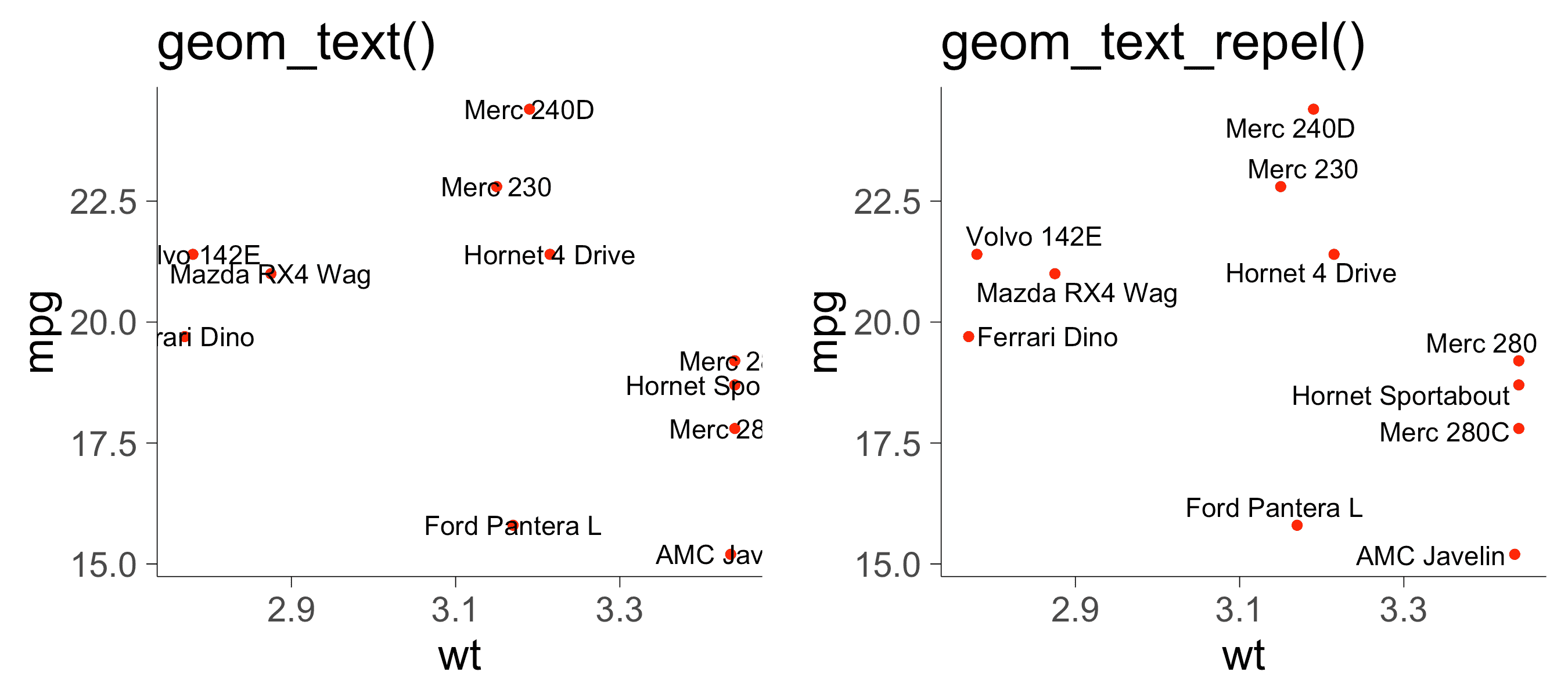Installation

ggrepel version 0.8.1 is available on CRAN:

``install.packages("ggrepel")``

The latest development version may have new features, and you can get it from GitHub:

``````# Use the devtools package
# install.packages("devtools")
devtools::install_github("slowkow/ggrepel")

# Or use the install-github.me service
source("https://install-github.me/slowkow/ggrepel")``````

Options

Options available for geom_text() and geom_label() are also available for `geom_text_repel()` and `geom_label_repel()`, including `size`, `angle`, `family`, `fontface`, etc.

ggrepel provides additional options for `geom_text_repel` and `geom_label_repel`:

Option Default Description
`force` `1` force of repulsion between overlapping text labels
`direction` `"both"` move text labels “both” (default), “x”, or “y” directions
`max.iter` `2000` maximum number of iterations to try to resolve overlaps
`nudge_x` `0` adjust the starting x position of the text label
`nudge_y` `0` adjust the starting y position of the text label
`box.padding` `0.25 lines` padding around the text label
`point.padding` `0 lines` padding around the labeled data point
`segment.color` `"black"` line segment color
`segment.size` `0.5 mm` line segment thickness
`segment.alpha` `1.0` line segment transparency
`arrow` `NULL` render line segment as an arrow with `grid::arrow()`

Examples

Hide some of the labels

Set labels to the empty string `""` to hide them. All data points repel the non-empty labels.

``````set.seed(42)

dat2 <- subset(mtcars, wt > 3 & wt < 4)
# Hide all of the text labels.
dat2\$car <- ""
# Let's just label these items.
ix_label <- c(2,3,16)
dat2\$car[ix_label] <- rownames(dat2)[ix_label]

ggplot(dat2, aes(wt, mpg, label = car)) +
geom_point(color = ifelse(dat2\$car == "", "grey50", "red")) +
geom_text_repel()``````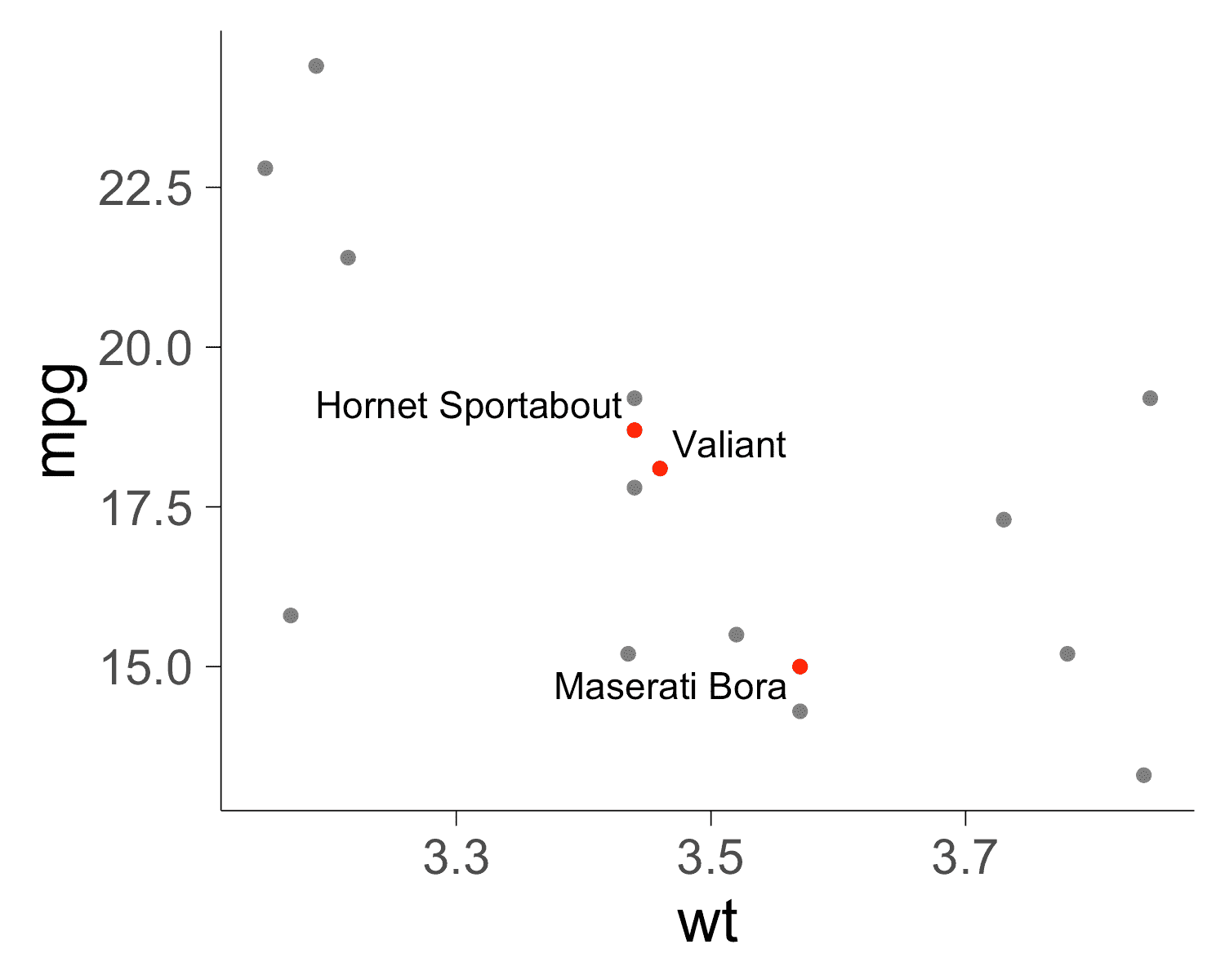Do not repel labels from data points

Set `point.padding = NA` to prevent label repulsion away from data points.

Now labels move away from each other and away from the edges of the plot.

``````set.seed(42)
ggplot(dat, aes(wt, mpg, label = car)) +
geom_point(color = "red") +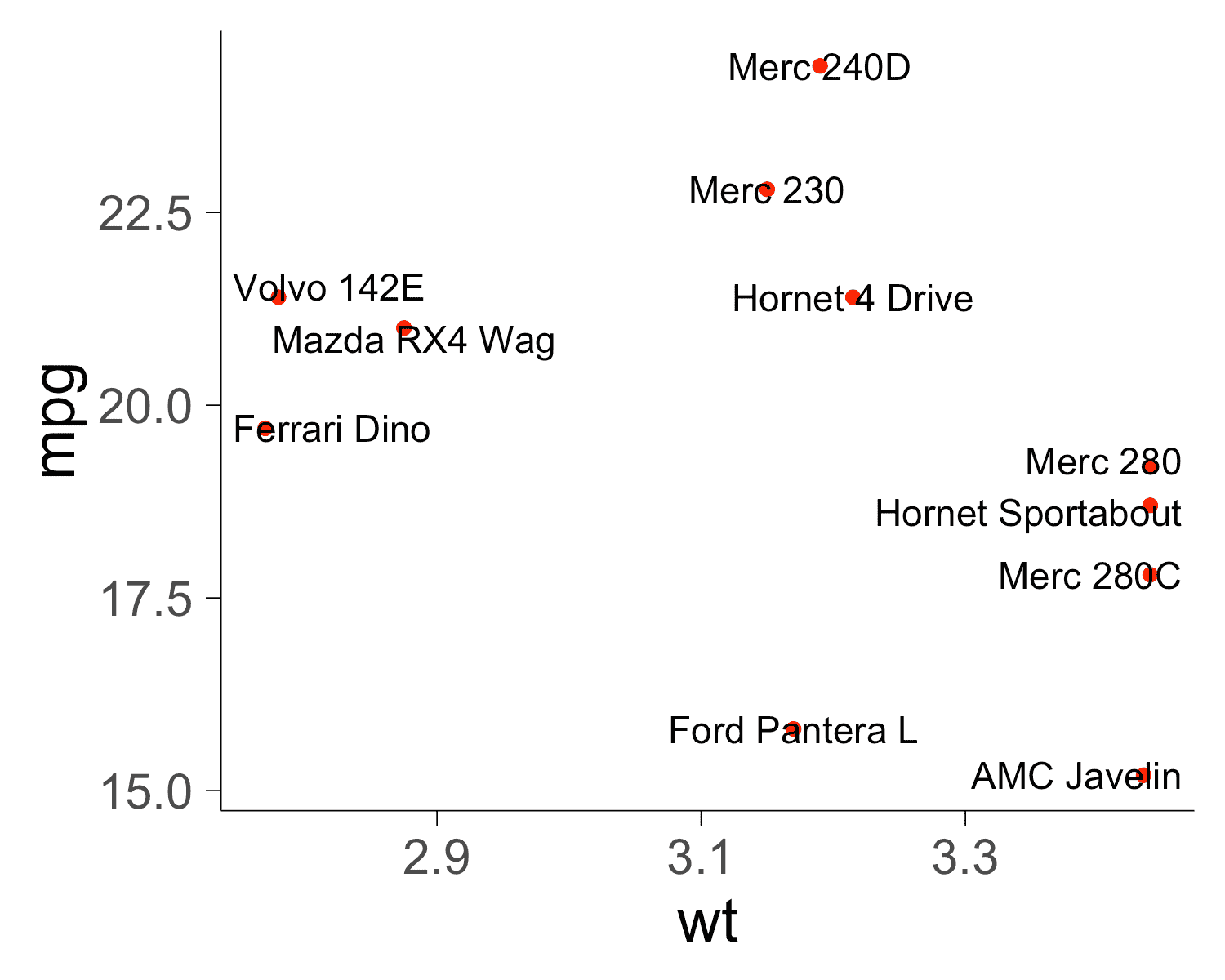Limit labels to a specific area

Use options `xlim` and `ylim` to constrain the labels to a specific area. Limits are specified in data coordinates. Use `NA` when there is no lower or upper bound in a particular direction.

Here we also use `grid::arrow()` to render the segments as arrows.

``````set.seed(42)

# All labels should be to the right of 3.
x_limits <- c(3, NA)

ggplot(dat, aes(wt, mpg, label = car, color = factor(cyl))) +
geom_vline(xintercept = x_limits, linetype = 3) +
geom_point() +
geom_label_repel(
arrow = arrow(length = unit(0.03, "npc"), type = "closed", ends = "first"),
force = 10,
xlim  = x_limits
) +
scale_color_discrete(name = "cyl")``````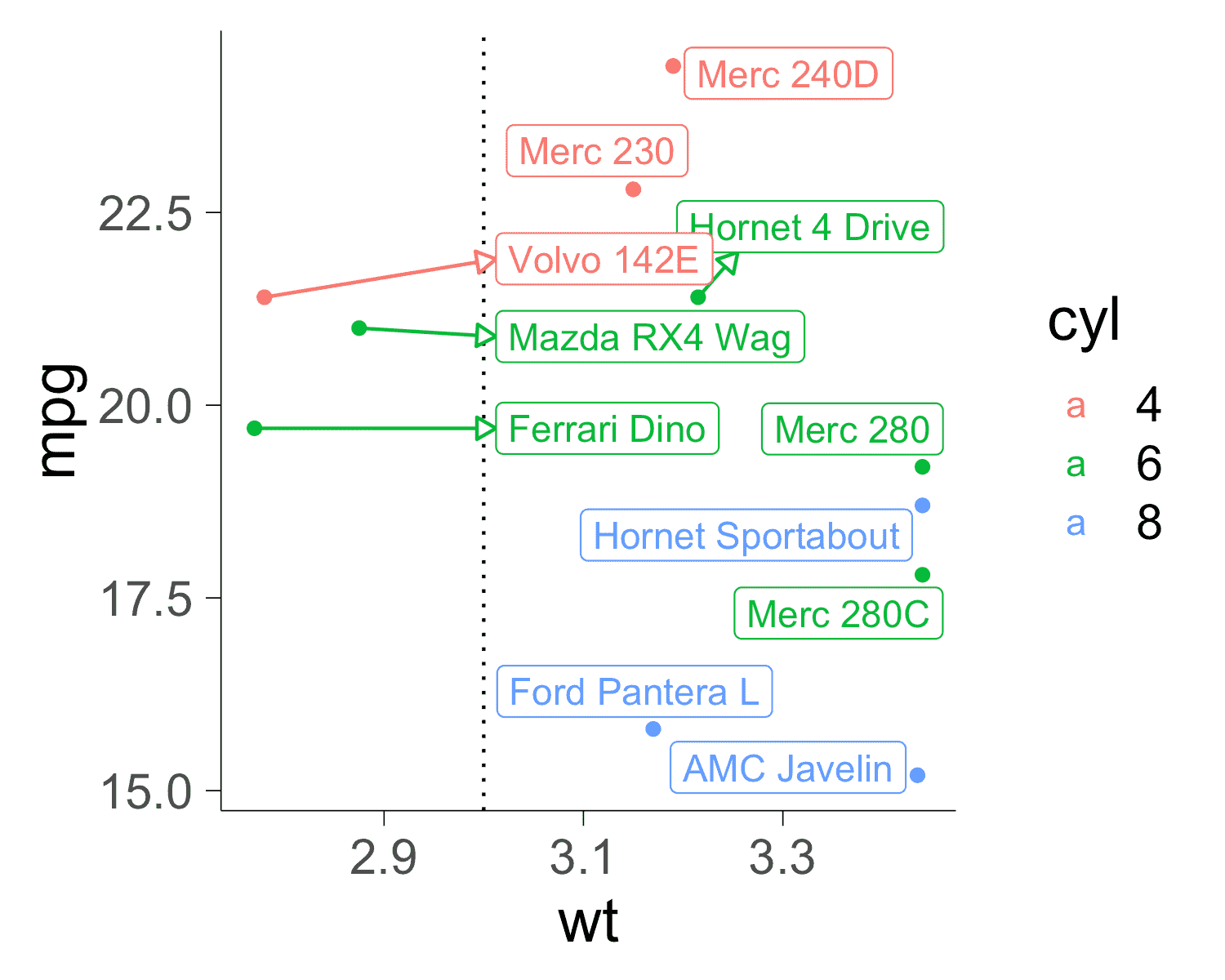Align labels on the top or bottom edge

Use `hjust` or `vjust` to justify the text neatly:

• `hjust = 0` for left-align
• `hjust = 0.5` for center
• `hjust = 1` for right-align

Sometimes the labels do not align perfectly. Try using `direction = "x"` to limit label movement to the x-axis (left and right) or `direction = "y"` to limit movement to the y-axis (up and down). The default is `direction = "both"`.

Also try using xlim() and ylim() to increase the size of the plotting area so all of the labels fit comfortably.

``````set.seed(42)

ggplot(mtcars, aes(x = wt, y = 1, label = rownames(mtcars))) +
geom_point(color = "red") +
geom_text_repel(
nudge_y      = 0.05,
direction    = "x",
angle        = 90,
vjust        = 0,
segment.size = 0.2
) +
xlim(1, 6) +
ylim(1, 0.8) +
theme(
axis.line.y  = element_blank(),
axis.ticks.y = element_blank(),
axis.text.y  = element_blank(),
axis.title.y = element_blank()
)``````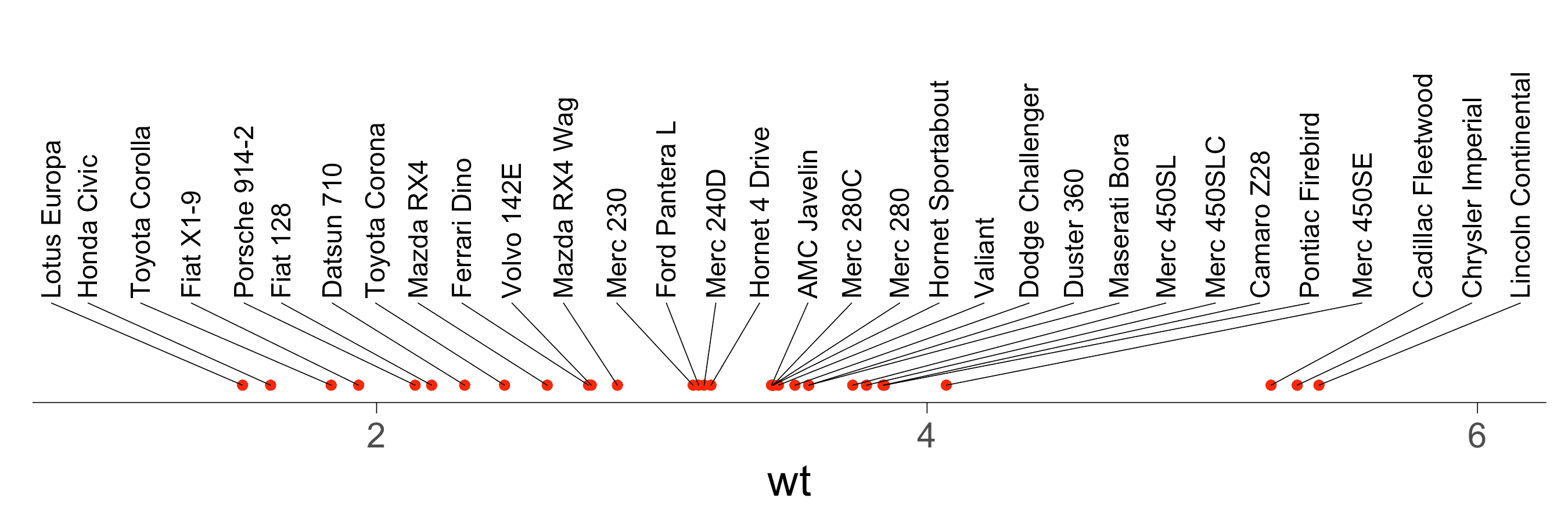Align text vertically with `nudge_y` and allow the labels to move horizontally with `direction = "x"`:

``````set.seed(42)

dat <- mtcars
dat\$car <- rownames(dat)

ggplot(dat, aes(qsec, mpg, label = car)) +
geom_text_repel(
data          = subset(dat, mpg > 30),
nudge_y       = 36 - subset(dat, mpg > 30)\$mpg,
segment.size  = 0.2,
segment.color = "grey50",
direction     = "x"
) +
geom_point(color = ifelse(dat\$mpg > 30, "red", "black")) +
scale_x_continuous(expand = c(0.05, 0.05)) +
scale_y_continuous(limits = c(NA, 36))``````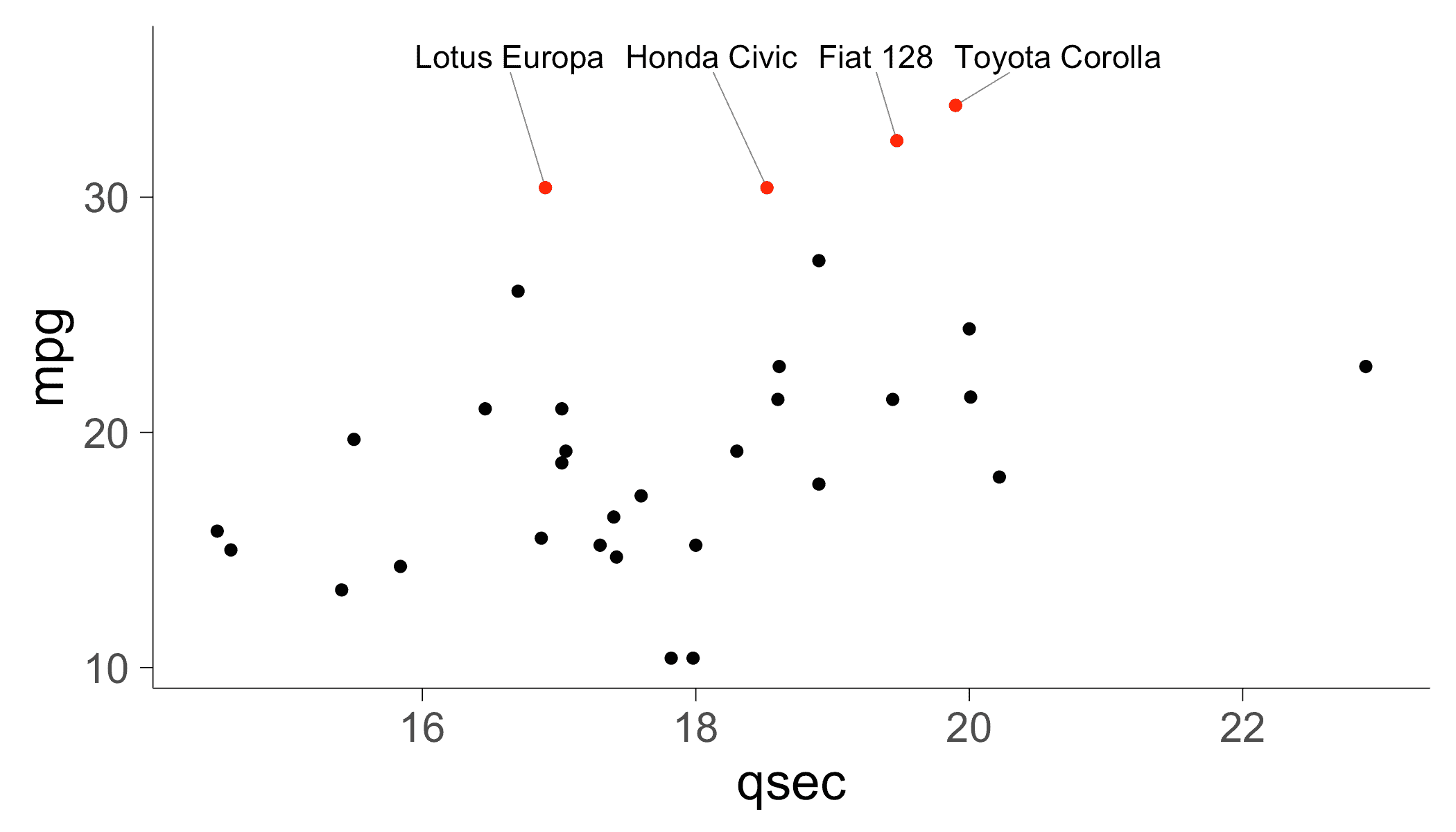Align labels on the left or right edge

Set `direction` to “y” and try `hjust` 0.5, 0, and 1:

``````set.seed(42)

p <- ggplot(mtcars, aes(y = wt, x = 1, label = rownames(mtcars))) +
geom_point(color = "red") +
ylim(1, 5.5) +
theme(
axis.line.x  = element_blank(),
axis.ticks.x = element_blank(),
axis.text.x  = element_blank(),
axis.title.x = element_blank(),
plot.title   = element_text(hjust = 0.5)
)

p1 <- p +
xlim(1, 1.375) +
geom_text_repel(
nudge_x      = 0.15,
direction    = "y",
hjust        = 0,
segment.size = 0.2
) +
ggtitle("hjust = 0")

p2 <- p +
xlim(1, 1.375) +
geom_text_repel(
nudge_x      = 0.2,
direction    = "y",
hjust        = 0.5,
segment.size = 0.2
) +
ggtitle("hjust = 0.5 (default)")

p3 <- p +
xlim(0.25, 1) +
scale_y_continuous(position = "right") +
geom_text_repel(
nudge_x      = -0.35,
direction    = "y",
hjust        = 1,
segment.size = 0.2
) +
ggtitle("hjust = 1")

gridExtra::grid.arrange(p1, p2, p3, ncol = 3)``````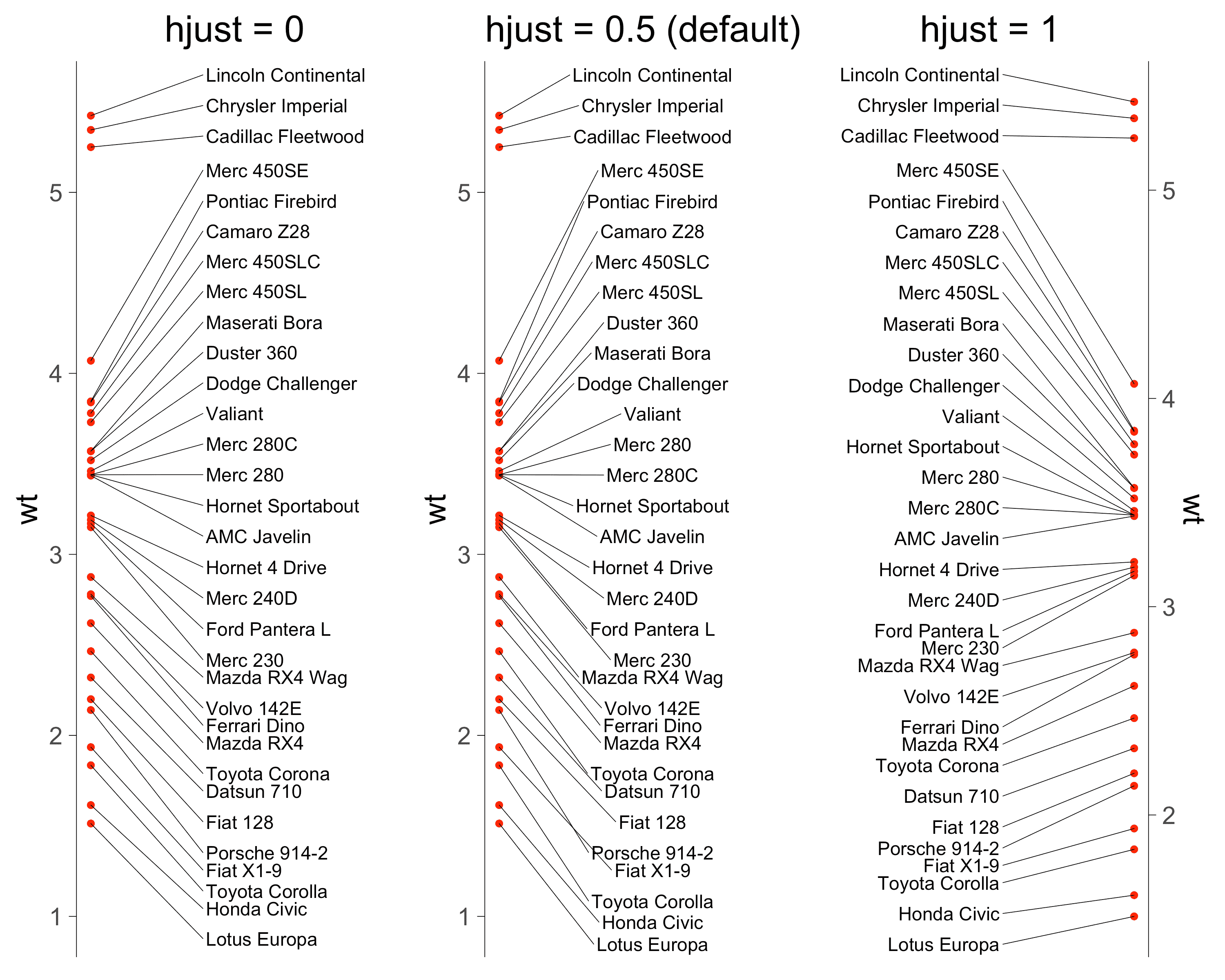Align text horizontally with `nudge_x` and `hjust`, and allow the labels to move vertically with `direction = "y"`:

``````set.seed(42)

dat <- subset(mtcars, wt > 2.75 & wt < 3.45)
dat\$car <- rownames(dat)

ggplot(dat, aes(wt, mpg, label = car)) +
geom_text_repel(
data          = subset(dat, wt > 3),
nudge_x       = 3.5 - subset(dat, wt > 3)\$wt,
segment.size  = 0.2,
segment.color = "grey50",
direction     = "y",
hjust         = 0
) +
geom_text_repel(
data          = subset(dat, wt < 3),
nudge_x       = 2.7 - subset(dat, wt < 3)\$wt,
segment.size  = 0.2,
segment.color = "grey50",
direction     = "y",
hjust         = 1
) +
scale_x_continuous(
breaks = c(2.5, 2.75, 3, 3.25, 3.5),
limits = c(2.4, 3.8)
) +
geom_point(color = "red")``````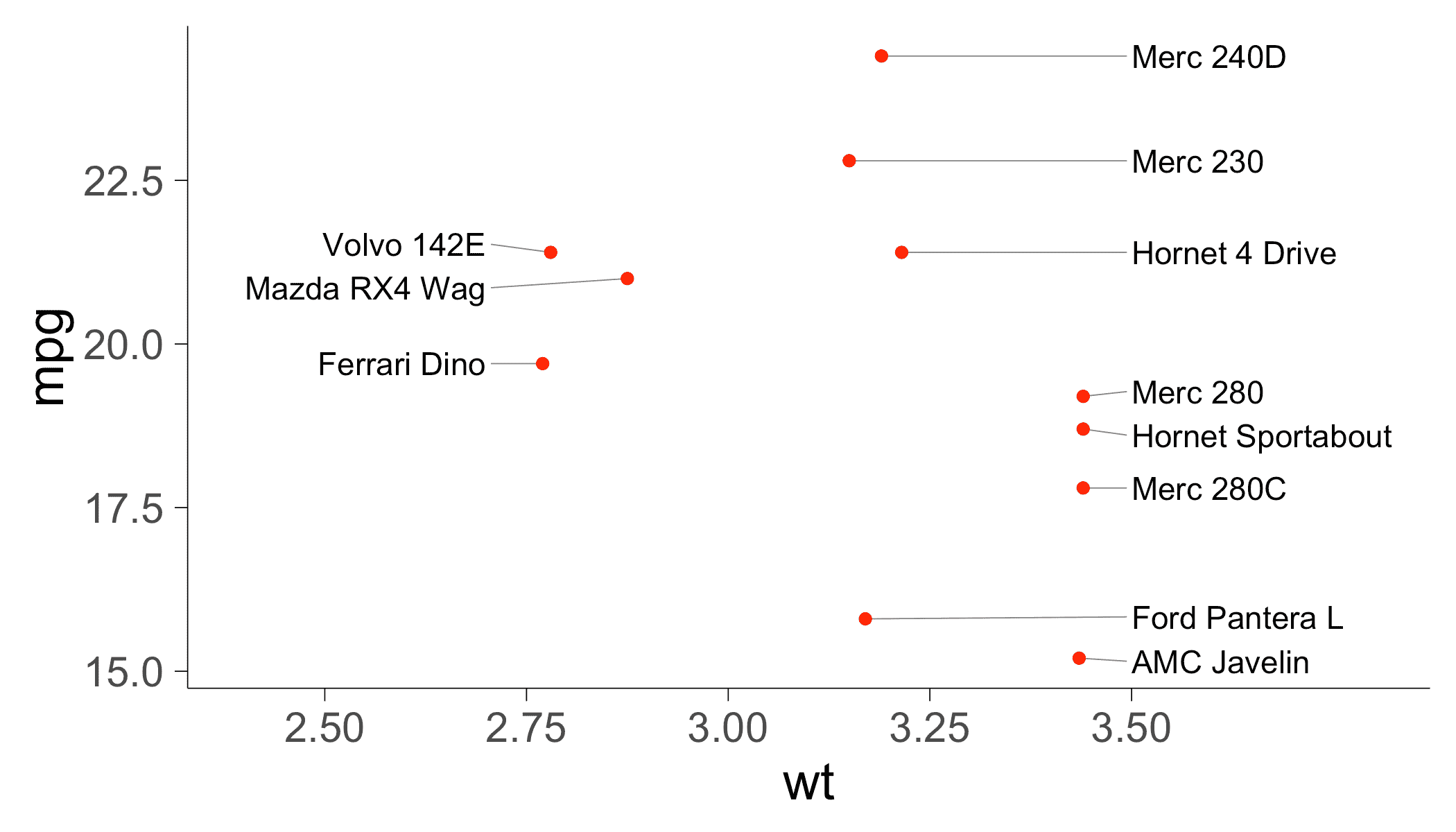Polar coordinates

``````set.seed(42)

mtcars\$label <- rownames(mtcars)
mtcars\$label[mtcars\$mpg < 25] <- ""

ggplot(mtcars, aes(x = wt, y = mpg, color = factor(cyl), label = label)) +
coord_polar(theta = "x") +
geom_point(size = 2) +
scale_color_discrete(name = "cyl") +
geom_text_repel(show.legend = FALSE) + # Don't display "a" in the legend.
theme_bw(base_size = 18)``````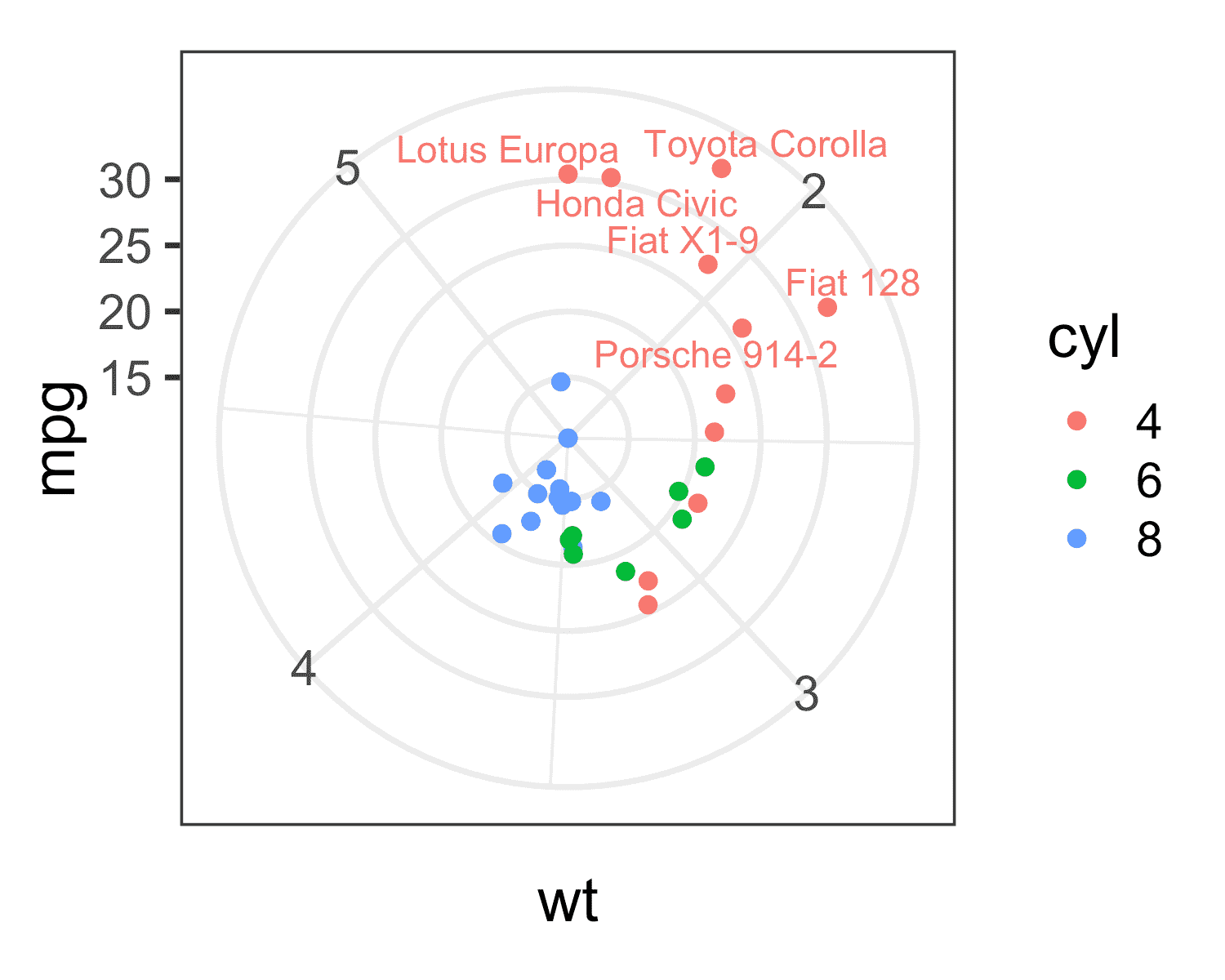Mathematical expressions

``````d <- data.frame(
x    = c(1, 2, 2, 1.75, 1.25),
y    = c(1, 3, 1, 2.65, 1.25),
math = c(
NA,
"integral(f(x) * dx, a, b)",
NA,
"lim(f(x), x %->% 0)",
NA
)
)

ggplot(d, aes(x, y, label = math)) +
geom_point() +
geom_label_repel(
parse       = TRUE, # Parse mathematical expressions.
size        = 8,
)``````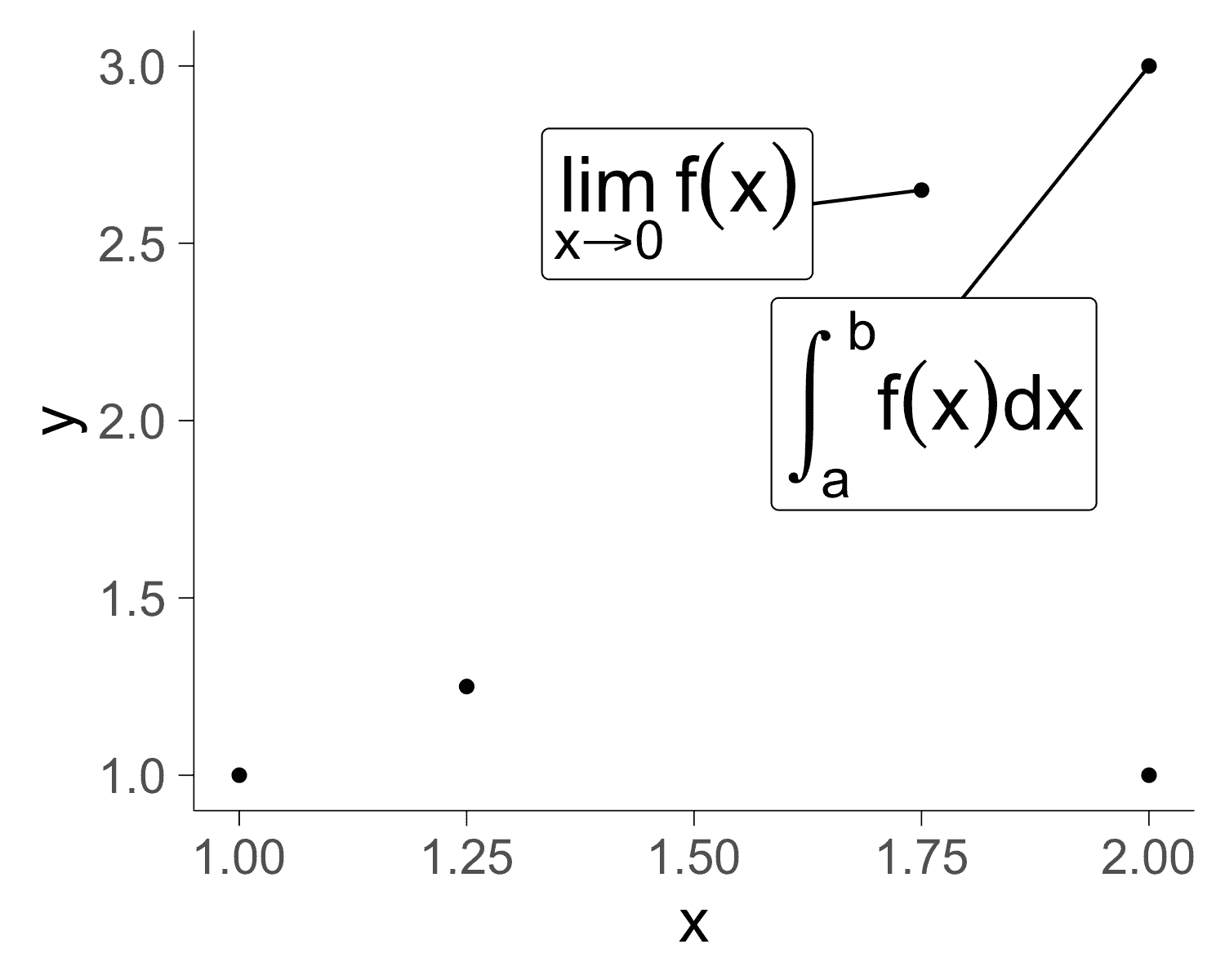Animation

``````# This chunk of code will take a minute or two to run.
library(ggrepel)
library(animation)

plot_frame <- function(n) {
set.seed(42)
p <- ggplot(mtcars, aes(wt, mpg, label = rownames(mtcars))) +
geom_point(color = "red") +
geom_text_repel(
size = 5, force = 3, max.iter = n
) +
theme_minimal(base_size = 16)
print(p)
}

saveGIF(
lapply(ceiling(1.75^(1:12)), function(i) {
plot_frame(i)
}),
interval   = 0.20,
ani.width  = 800,
ani.heigth = 600,
movie.name = "animated.gif"
)``````

Source code

View the source code for this vignette on GitHub.

R Session Info

``sessionInfo()``
``````## R version 3.5.1 (2018-07-02)
## Platform: x86_64-apple-darwin15.6.0 (64-bit)
## Running under: macOS High Sierra 10.13.6
##
## Matrix products: default
## BLAS: /Library/Frameworks/R.framework/Versions/3.5/Resources/lib/libRblas.0.dylib
## LAPACK: /Library/Frameworks/R.framework/Versions/3.5/Resources/lib/libRlapack.dylib
##
## locale:
##  C/en_US.UTF-8/en_US.UTF-8/C/en_US.UTF-8/en_US.UTF-8
##
## attached base packages:
##  stats     graphics  grDevices utils     datasets  methods   base
##
## other attached packages:
##  ggrepel_0.8.1 ggplot2_3.1.0 gridExtra_2.3 knitr_1.21
##
## loaded via a namespace (and not attached):
##   Rcpp_1.0.1       magrittr_1.5     tidyselect_0.2.5 munsell_0.5.0
##   colorspace_1.4-1 R6_2.4.0         rlang_0.3.1.9000 dplyr_0.8.0.9002
##   stringr_1.4.0    plyr_1.8.4       tools_3.5.1      grid_3.5.1
##  gtable_0.3.0     xfun_0.5         withr_2.1.2      htmltools_0.3.6
##  assertthat_0.2.1 yaml_2.2.0       lazyeval_0.2.2   digest_0.6.18
##  tibble_2.1.1     crayon_1.3.4     purrr_0.3.0      codetools_0.2-16
##  prettydoc_0.2.1  glue_1.3.1       evaluate_0.13    rmarkdown_1.11
##  labeling_0.3     stringi_1.4.3    compiler_3.5.1   pillar_1.3.1
##  scales_1.0.0     pkgconfig_2.0.2``````